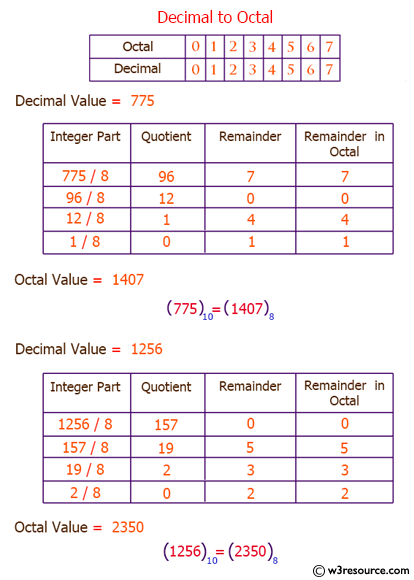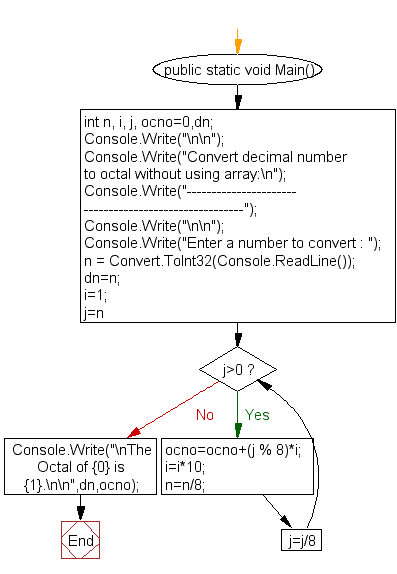﻿ C#: Convert decimal number to octal without using an array# C# Sharp Exercises: Convert decimal number to octal without using an array

## C# Sharp For Loop: Exercise-50 with Solution

Write a program in C# Sharp to convert a decimal number into octal without an using an array.

Pictorial Presentation:Sample Solution:

C# Sharp Code:

``````using System;
public class Exercise50
{
public static void Main()
{
int n, i, j, ocno=0,dn;

Console.Write("\n\n");
Console.Write("Convert decimal number to octal without using array:\n");
Console.Write("------------------------------------------------------");
Console.Write("\n\n");

Console.Write("Enter a number to convert : ");
dn=n;
i=1;

for(j=n;j>0;j=j/8)
{
ocno=ocno+(j % 8)*i;
i=i*10;
n=n/8;
}

Console.Write("\nThe Octal of {0} is {1}.\n\n",dn,ocno);
}
}
```
```

Sample Output:

```Convert decimal number to octal without using array:
------------------------------------------------------
Enter a number to convert : 6
The Octal of 6 is 6.
```

Flowchart:C# Sharp Code Editor:

Contribute your code and comments through Disqus.

What is the difficulty level of this exercise?

Test your Programming skills with w3resource's quiz.

﻿